Miscellaneous Test: Time & Work- 2

# Miscellaneous Test: Time & Work- 2

Test Description

## 25 Questions MCQ Test IBPS PO Prelims- Study Material, Online Tests, Previous Year | Miscellaneous Test: Time & Work- 2

Miscellaneous Test: Time & Work- 2 for Banking Exams 2023 is part of IBPS PO Prelims- Study Material, Online Tests, Previous Year preparation. The Miscellaneous Test: Time & Work- 2 questions and answers have been prepared according to the Banking Exams exam syllabus.The Miscellaneous Test: Time & Work- 2 MCQs are made for Banking Exams 2023 Exam. Find important definitions, questions, notes, meanings, examples, exercises, MCQs and online tests for Miscellaneous Test: Time & Work- 2 below.
Solutions of Miscellaneous Test: Time & Work- 2 questions in English are available as part of our IBPS PO Prelims- Study Material, Online Tests, Previous Year for Banking Exams & Miscellaneous Test: Time & Work- 2 solutions in Hindi for IBPS PO Prelims- Study Material, Online Tests, Previous Year course. Download more important topics, notes, lectures and mock test series for Banking Exams Exam by signing up for free. Attempt Miscellaneous Test: Time & Work- 2 | 25 questions in 40 minutes | Mock test for Banking Exams preparation | Free important questions MCQ to study IBPS PO Prelims- Study Material, Online Tests, Previous Year for Banking Exams Exam | Download free PDF with solutions
 1 Crore+ students have signed up on EduRev. Have you?
Miscellaneous Test: Time & Work- 2 - Question 1

### X Can finish a piece of work in 12 days while Y can do it in 15 days. If both work at it together. How much time will they take to do the work?

Detailed Solution for Miscellaneous Test: Time & Work- 2 - Question 1

Efficiency of A = 100 / 12 = 8.33%
Efficiency of B = 100 / 15 = 6.66%
Combined efficiency of A and B = 8.33 + 6.66 = 15%
Number of days taken by A and B, when working together = 100 / 15 =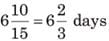Note : Efficiency x Time period = Fixed amount of work
Also, in terms of percentage total work to be done is considered as 100% (in fraction it is 1), unless otherwise stated.
Alternatively : It can be done through unitary method, also.

Miscellaneous Test: Time & Work- 2 - Question 2

### X can do piece of work in 5 days. Y can do it in 10 days. With the help of Z, they finish the work in 2 days. In how many days Z alone can do the whole work?

Detailed Solution for Miscellaneous Test: Time & Work- 2 - Question 2

►Efficiency of A = 20% = 100 / 5

►Efficiency of B = 10% = 100 / 10

►Efficiency of A,B and C = 50%

►∴ Efficiency of C = (Efficiency of A,B and C) – (Efficiency of A and B) = (50) - (20 + 10) = 20%

►∴ Number of days required by C to work alone = 100 / 20 = 5 days

Alternatively: Go through options and satisfy the values.

Miscellaneous Test: Time & Work- 2 - Question 3

### A is thrice as good a work man as B and takes 10 days less to do a piece of work than B takes. The number of days taken by B to finish the work is:

Detailed Solution for Miscellaneous Test: Time & Work- 2 - Question 3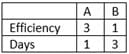(Since number of days are inversely proportional to the efficiency)
Now if A requires x days, so B requires 3x days.
∴ Difference of required days = (3x - x) = 2x = 10
► ⇒ x = 5
Hence the number of days required by
► B = 3x = 3 x 5 = 15 days

Miscellaneous Test: Time & Work- 2 - Question 4

Ajit is 3 times as efficient as Bablu, then the ratio of number of days required by each to work alone, completely?

Detailed Solution for Miscellaneous Test: Time & Work- 2 - Question 4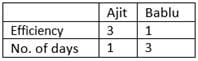Miscellaneous Test: Time & Work- 2 - Question 5

A is thrice as efficient as B. Working together they complete the work in 3 days. If B takes 8 days more than A, what is the number of days taken by A to finish the whole work, alone?

Detailed Solution for Miscellaneous Test: Time & Work- 2 - Question 5

Efficiency of A + B = 33.33% = (100 / 3)

Ratio of efficiency of A and B = 3 : 1
∴ Efficiency of A =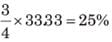∴ Number of days taken by A = 4 = 100 /25
Alternatively :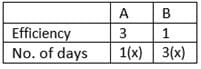∴ Difference in days = 2x = 8
► ⇒ x = 4 and 3x = 12

Therefore number of days taken by A, working alone = 4 days
Alternatively :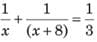Now, you can use the options, or solve the equations to get the value of x which is equal to 4.

Miscellaneous Test: Time & Work- 2 - Question 6

Ganga, Jamuna and Saraswati can do a piece of work, working together, in 1 day. Ganga is thrice efficient as Jamuna and Jamuna takes the twice the number of days as Saraswati takes to do it alone. What is the difference between the number of days taken by Ganga and Saraswati?

Detailed Solution for Miscellaneous Test: Time & Work- 2 - Question 6

Remember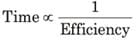Now,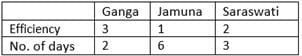Again, efficiency of Ganga, Jamuna and Saraswati
= 100 / 1 = 100%
∴ Efficiency of Ganga =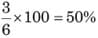Efficiency of Saraswati =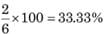Now, number of days taken by Ganga
= 100 / 50 = 2
Number of days taken by Saraswati
= 100 / 33.33 = 3
∴ Difference in number of days taken by Ganga and Saraswati
= 3 - 2 = 1 day

Miscellaneous Test: Time & Work- 2 - Question 7

A and B can complete a task in 30 days when working together. After A and B have been working together for 11 days, B is called away and A, all by himself completes the task in the next 28 days. Had A been working alone from begining, the number of days taken by him to complete the task would have been:

Detailed Solution for Miscellaneous Test: Time & Work- 2 - Question 7

Work done in 11 days = 11 / 30
Rest work = 19 / 30
1 day's work of A =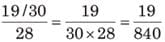Total number of days required to complete the whole work alone.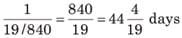Miscellaneous Test: Time & Work- 2 - Question 8

Efficiency of Asha is 25% more than Usha and Usha takes 25 days to complete a piece of work, Asha started a work alone and then Usha joined her 5 days before actual completion of the work. For how many days Asha worked alone?

Detailed Solution for Miscellaneous Test: Time & Work- 2 - Question 8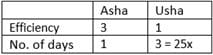∴ Number of days required by Asha to finish the work alone = 20
► ∴ (4 x = 4 x 5)
(Alternatively, from percentage change graphic, number of days taken by Asha will be 20% less than Usha, if efficiency of Asha is 25% more than Usha)
Now, since Asha and Usha did work together for last 5 days = 5 x 9 = 45%
(Since efficiency of Asha = 5% and Usha's efficiency = 4%)
It means Asha completed 55% work alone
∴ Number of days taken by Asha to complete 55% work = 55 / 5 = 11

Miscellaneous Test: Time & Work- 2 - Question 9

Chandni and Divakar can do a piece of work in 9 days and 12 days respectively. If they work for a day alternatively, Chandni beginning, in how many days, the work will be completed?

Detailed Solution for Miscellaneous Test: Time & Work- 2 - Question 9

Efficiency of Chandni = 11.11%
Efficiency of Divakar = 8.33%
They do 19.44% work in 2 days.
∴ They need 10 days to do 97.22% work,
Now the rest work (2.78) was done by Chandni in 2.78 / 11.11 = 1 / 4 days
Therefore total number of days required =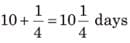Alternatively :
Chandni's one day's work = 1 / 9
Divakar's one day's work = 1 / 12
Chandni's and Divakar's (1 + 1) = 2 day's work = 1 / 9 + 1 / 12 = 7 / 36
So, in 10 days they do =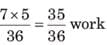So, the remaining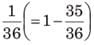work will be done by Chandni =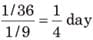Thus total number of required days =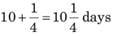Hint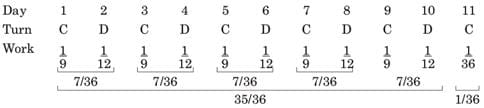Miscellaneous Test: Time & Work- 2 - Question 10

A group of men decided to do a job in 4 days. 20 men dropped out from the task every day due to which work was completed in 7 days. How many men were there at the beginning?

Detailed Solution for Miscellaneous Test: Time & Work- 2 - Question 10

Let X be the initial number of men then,
According to the question,
4X = X + (X - 20) + (X - 40) + (X - 60) + (X - 80) + (X - 100) + (X - 120)
⇒ 4X = 7X - 420
⇒ 3X = 420
⇒ X = 420/3
⇒ X = 140 men

Miscellaneous Test: Time & Work- 2 - Question 11

The number of days required by A,B and C to work individually is 6, 12 and 8 respectively. They started a work doing it alternatively. If A has started then followed by B and so on, how many days are needed to complete the whole work?

Detailed Solution for Miscellaneous Test: Time & Work- 2 - Question 11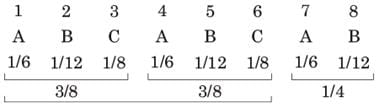► 6 / 8 = 3 / 4
In 3 days A,B,C do 3 / 8 work,
In 6 days A,B,C do 3 / 4 work
Rest work = 1 / 4, which is less than 3 / 8, On the 7th day, 1 / 6 more work will be done by A
Now rest work =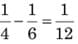Now, this rest work (1 / 12) will be done by B in 1 complete day.
Thus, total number of days = 6 + 1 + 1 = 8 days.
Alternatively : Efficiency of A = 16.66% , Efficiency of B = 8.33% , Efficiency of C = 12.5%
Efficiency of A + B = 25% , Efficiency of A + B + C = 37.5%
In 3 days A,B,C completes 37.5% work, In 6 days A,B,C completes 75% work
Rest work = 25%
This 25% work will be completed by A and B in next 2 days, Thus total 6 + 2 = 8 days are needed.

Miscellaneous Test: Time & Work- 2 - Question 12

When A,B and C are deployed for a task, A and B together do 70% of the work and B and C together do 50% of the work. Who is most efficient?

Detailed Solution for Miscellaneous Test: Time & Work- 2 - Question 12

A + B = 70%
B + C = 50%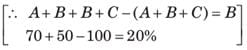⇒ B = 20% , A = 50% and C = 30%
Hence, A is most efficient.

Miscellaneous Test: Time & Work- 2 - Question 13

Sharma is 20% less efficient than Kelkar. If Kelkar can do a piece of work in 24 days. the number of days required by Sharma to complete the same work alone will be—

Detailed Solution for Miscellaneous Test: Time & Work- 2 - Question 13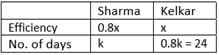► ∴ 0.8k = 24 ⇒ k = 30
Thus Sharma requires 30 days, to complete the work, alone.

Miscellaneous Test: Time & Work- 2 - Question 14

If 10 persons can do a job in 20 days, then 20 person with twice the efficiency can do the same job in:

Detailed Solution for Miscellaneous Test: Time & Work- 2 - Question 14

M x D = 10 x 20 = 200
Man-days New Man-days = (20 x 2) x x
► 200 = 20 x 2 x x
► x = 5 days
or M1D1 = M2D2
► 10 x 20 = (20 x 2) x x
► ⇒ x = 5

Miscellaneous Test: Time & Work- 2 - Question 15

30 workers can finish a work in 20 days. After how many days should 9 workers leave the job so that the work is completed in total 26 days :

Detailed Solution for Miscellaneous Test: Time & Work- 2 - Question 15

Go through options. Consider option (c)
► 30 x 20 = 30 x 6 + 21 x 20
► 600 = 600,
Hence presumed option is correct.

Alternatively :
► ​30 x 20 = 30 x x + 21 x (26 - x )
► ⇒ x = 6

Miscellaneous Test: Time & Work- 2 - Question 16

Mr. Modi can copy 40 pages in 10 minutes, Mr. Xerox and Mr. Modi both working together can copy 250 in 25 minutes. In how many minutes Mr. Xerox can copy 36 pages?

Detailed Solution for Miscellaneous Test: Time & Work- 2 - Question 16

Efficiency (per minute) of Modi = 4 copies/min
Efficiency of Modi and Xerox together = 10 pages/min
∴ Efficiency of Xerox alone = 10 - 4 = 6 pages/min
∴ Mr. Xerox needs 6 minutes to copy 36 pages.

Miscellaneous Test: Time & Work- 2 - Question 17

6 children and 2 men complete a certain piece of work in 6 days. Each child takes twice the time taken by a man to finish the work. In how many days will 5 men finish the same work?

Detailed Solution for Miscellaneous Test: Time & Work- 2 - Question 17

6C + 2 M = 6 days
► ⇒ 36C + 12 M = 1 day
Again 1M = 2C
► ∴ 36 + 12 x 2 = 1 day
60 children can do the work in 1 day Now,
► 5 men = 10 children
∴ 10 children can do the work in 6 days.

Miscellaneous Test: Time & Work- 2 - Question 18

Directions for question : A can do a work in 15 days and B can do it in 18 days. with the help of C work is completed in 6 days.

Q. How long will it take for C to finish the work alone?

Detailed Solution for Miscellaneous Test: Time & Work- 2 - Question 18

Efficiency of A = 6.66% , Efficiency of B = 5.55% , Efficiency of A + B + C = 16.66%
∴ Efficiency of C = 4.44%
Now, number of days required by C =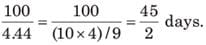Miscellaneous Test: Time & Work- 2 - Question 19

Directions for question : A can do a work in 15 days and B can do it in 18 days. with the help of C work is completed in 6 days.

Q. A,B and C received total ₹ 27,000 for the whole work. What is the share of B, if the money is distributed in the ratio of amount of work done, individually?

Detailed Solution for Miscellaneous Test: Time & Work- 2 - Question 19

Explanation : A = 15 days

B = 18 days

C = 6 days

Taking LCM, we get 180

Efficiency of A = 12

Efficiency of B = 10

Efficiency of A+B+C = 6

A.T.Q

=> (10/30)*27000

= 9000

Miscellaneous Test: Time & Work- 2 - Question 20

314 weavers weaves 6594 shawls in 1 / 6 hours. What is the number of shawls weaved per hour by an average weaver?

Detailed Solution for Miscellaneous Test: Time & Work- 2 - Question 20

In 1 hour 314 weavers weave = 6594 x 6
shawls In 1 hour 1 weavers weave  =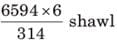= 126 shawls

Miscellaneous Test: Time & Work- 2 - Question 21

If 20 engineers and 20 workers can together construct a 20 km road in 20 days. 40 engineers and 40 workers together will construct 40 km road in how many days?

Detailed Solution for Miscellaneous Test: Time & Work- 2 - Question 21

Equate the man-days
For 20 km road, 20 x 20 = 400 man-days are required
∴ For 40 km road 800 man-days are required So, 800 = 40 * x
► ⇒ x = 20

Miscellaneous Test: Time & Work- 2 - Question 22

A man, a woman and a girl worked for a contractor for the same period. A man is twice efficient as a woman and a woman is thrice efficient as a girl. ₹ 10000 were given to all of them. What is the sum of money received by a woman and a girl together?

Detailed Solution for Miscellaneous Test: Time & Work- 2 - Question 22

Efficiency of a man : woman : girl = 6 : 3 : 1
∴ Share of a woman and girl =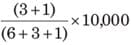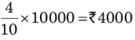Miscellaneous Test: Time & Work- 2 - Question 23

There was a leakage in the container of the refined oil. If 11 kg oil leaks out per day then it would have lasted for 50 days, if the leakage was 15 kg per day, then it would have lasted for only 45 days. For how many days would the oil have lasted, if there was no leakage and it was completely used for eating purpose?

Detailed Solution for Miscellaneous Test: Time & Work- 2 - Question 23

Let x kg of oil is used for eating purpose, daily, then
► (x + 11) x 50 = + 15 x 45
► x = 25
∴ Total quantity of oil = (25 + 11) x 50 = 1800
∴ Required number of days = 1800 / 25 = 72

Miscellaneous Test: Time & Work- 2 - Question 24

Tap A can fill a tank in 20 hours, B in 25 hours but tap C can empty a full tank in 30 hours. Starting with A, followed by B and C each tap opens alternatively for one hour period till the tank gets filled up completely. In how many hours the tank will be filled up completely?

Detailed Solution for Miscellaneous Test: Time & Work- 2 - Question 24

Explanation : LCM(20,25,30)=300

Suppose capacity of the tank is 300 litre.

Quantity filled by tap A in 1 hour

= 300/20

= 15 litre.

Quantity filled by tap B in 1 hour = 300/25

= 12 litre.

Quantity emptied by tap C in 1 hour = 300/30

= 10 litre.

In every three hour, 15 + 12 − 10 = 17 litre is filled.

In 45 hours,

17 × 15 = 255 litre is filled.

Remaining quantity to be filled = 300 − 255 = 45 litre.

In next 3 hour, 17 more litre is filled.

Remaining quantity to be filled = 45 − 17 = 28 litre.

In next 2 hour hour, 15 + 12 = 27 more litre is filled.

Remaining quantity to be filled = 28 − 27 = 1 litre.

But in next 1 hour, 10 litre is emptied.

Remaining quantity to be filled = 1 + 10

= 11 litre.

Pipe A fills this remaining quantity in 11/15hour (i.e, in 44 minutes).

Therefore, the tank is filled in 51 hour 44 minutes

Miscellaneous Test: Time & Work- 2 - Question 25

Pipe A can fill the tank in 4 hours, while pipe B can fill it in 6 hours working separately. Pipe C can empty the whole tank in 4 hours. Ramesh opened the pipe A and B simultaneously to fill the empty tank. He wanted to adjust his alarm so that he could open the pipe C when it was half-filled, but he mistakenly adjusted his alarm at a time when his tank would be 3/4th filled. What is the time difference between both the cases, to fill the tank fully.

Detailed Solution for Miscellaneous Test: Time & Work- 2 - Question 25

In ideal case :
Time taken to fill the half tank by A and B = 50 / 41.66 = 6 / 5 hours
Time taken by A,B and C to fill rest half of the tank = 50 / 16.66 = 3 hours
Total time =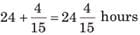In second case :
Time taken to fill 3 / 4 tank by A and B = 75 / 41.66 = 9 / 5 hours
Time taken by A,B and C to fill rest 1 / 4 tank = 25 / 16.66 = 3 / 2 hours
Total time = 9 / 5 + 3 / 2 = 3 hours 18 minutes
Therefore, difference in time = 54 minutes

## IBPS PO Prelims- Study Material, Online Tests, Previous Year

173 videos|192 docs|149 tests
 Use Code STAYHOME200 and get INR 200 additional OFF Use Coupon Code
Information about Miscellaneous Test: Time & Work- 2 Page
In this test you can find the Exam questions for Miscellaneous Test: Time & Work- 2 solved & explained in the simplest way possible. Besides giving Questions and answers for Miscellaneous Test: Time & Work- 2, EduRev gives you an ample number of Online tests for practice

## IBPS PO Prelims- Study Material, Online Tests, Previous Year

173 videos|192 docs|149 tests